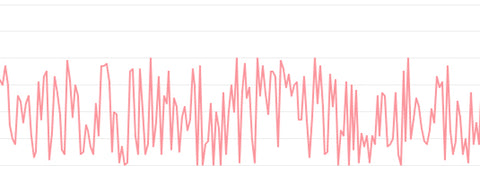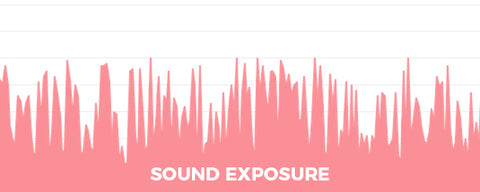Leq is one of the most important variables to be calculated when complying with regulations such as the Noise at Work Regulations (2005). Leq is the Equivalent Continuous Sound Level and represents sound exposure over an elapsed period of time. But getting to this value requires multiple steps and the use of what is known as an Integrating Sound Level Meter. These meters normally do all the hard work behind the scenes to give you a nice easy Leq reading. Explaining Leq requires explaining various other sound measurements along the way – how convenient! Here is how it’s done…

## Calculating Leq

A sound meter responds to change in air pressure caused by sound waves; this is normally recorded in Pascals and conveniently converted into decibels (dB) for you behind the scenes. This is where the term ‘Sound Pressure Level’ (SPL) originates. Imagine plotting these dB points on a line graph, you would get something that looks a little like this.The area underneath the plotted points is representative of all of the sound energy that existed during this period. If it helps, image this area as the total sound that entered your ear. An integrating sound meter is designed specifically to be able to measure the total area of this section of the graph and as a result tell us the total amount of sound energy recorded. This is known as the ‘Sound Exposure’ and is measured in Pascal2 p/Hour (Pa2Hr). This is normally converted behind the scenes into dB’s again to give you the ‘Sound Exposure Level’ (SEL) or LE .An Leq measurement is now obtainable. By dividing the sound exposure by the time over which the measurement was taken we are given an average value of exposure for the period. This is known as Leq or Equivalent Continuous Sound Level.Sound meters that measure Leq are often referred to as both ‘Integrating’ and ‘Integrating-Averaging’ Sound Level Meters. Whilst the later term is the most technically correct, the two terms are often used interchangeably.